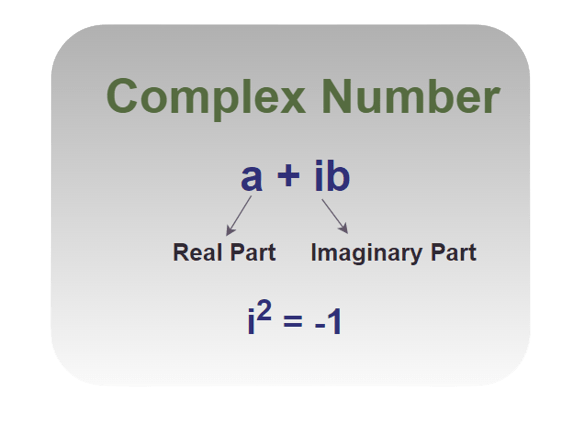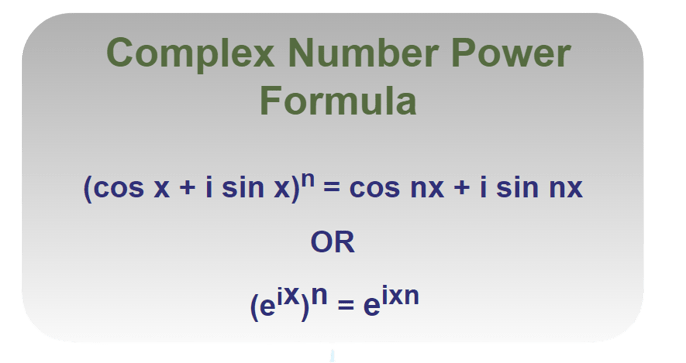Open In App
Related Articles
• Basic Math Formulas

# Complex Number Power Formula

Complex Numbers are numbers that can be written as a + ib, where a and b are real numbers and i (iota) is the imaginary component and its value is √(-1), and are often represented in rectangle or standard form. 10 + 5i, for example, is a complex number in which 10 represents the real component and 5i represents the imaginary part. Depending on the values of a and b, they might be wholly real or purely fictitious. When a = 0 in a + ib, ib is a totally imaginary number, and when b = 0, we get a, which is a strictly real number.

In this article, we will learn about, complex number power formulas, their examples, and others in detail.

## Complex Number Definition

A number that is written in the form of a+ib where i is an imaginary term and a and b are real numbers is called a Complex Number. The value of i is √-1. A complex number has two parts, Real Part and Imaginary Part. The real part consists of the real number ‘a’ while ‘ib’ is the imaginary part.## Complex Number Power Formula

To expand a complex number according to its specified exponent, it must first be transformed to its polar form, which has the modulus and argument as components. After that, De Moivre’s theorem is applied, which states:

De Moivre’s Formula states that for all real values of a number, say x,

(cos x + i sin x)n = cos (nx) + i sin (nx)

where n is any integer

or it can be written as

(eix)n = eixn## Complex Number Power Formula Derivation

DeMoivre’s Theorem can be derived with the help of Mathematical Induction as follows:

P(n): (cos x + i sin x)n = cos(nx) + isin(nx) ⇢ (1)

For n = 1, we have

P(1) = (cos x + i sin x)1

P(1) = cos(1x) + i sin(1x)

P(1) = cos(x) + i sin(x)

That is true and thus, P(1) is true.

Assuming P(k) is true, i.e.

P(k) = (cos x + i sin x)k = cos(kx) + i sin(kx) ⇢  (2)

Now, we just have to prove that the P(k+1) is also true.

P(k+1) = (cos x + i sin x)k+1

P(k+1) = (cos x + i sin x)k (cos x + i sin x)

P(k+1) = (cos (kx) + i sin (kx)) (cos x + i sin x) [Using (i)]

P(k+1) = cos (kx) cos x − sin(kx) sinx + i (sin(kx) cosx + cos(kx) sinx)

P(k+1) = cos {(k + 1)x} + i sin {(k + 1)x}

P(k+1) = (cos x + i sin x)k+1 = cos {(k + 1)x} + i sin {(k + 1)x}

Thus, P(k+1) is also true, thus by the principal of mathematical induction, P(n) is true.

Hence the result is proved.

## Solved Example on Complex Number Power Formula

Example 1: Expand (1 + i)5.

Solution:

Given,

• r = √(12 + 12) = √2
• θ = π/4

Polar form of (1 + i)According to De Moivre’s Theorem

(cosθ + sinθ)n = cos(nθ) + i sin(nθ)

Thus,

(1 + i)5= −4 − 4i

Example 2: Expand (2 + 2i)6.

Solution:

Here, r =, θ = π/4

The polar form of  (2 + 2i) =According to De Moivre’s Theorem: (cosθ + sinθ)n = cos(nθ) + i sin(nθ).

Thus, (2 + 2i)6= 512 (-i)

= −512i

Example 3: Expand (1 + i)18.

Solution:

Here, r =, θ = π/4

The polar form of (1+i) =According to De Moivre’s Theorem: (cosθ + sinθ)n = cos(nθ) + isin(nθ).

Thus, (1 + i)18= 512i

Example 4: Expand (-√3 + 3i)31.

Solution:

Here, r =, θ = 2π/3

The polar form of  (-√3 + 3i) =According to De Moivre’s Theorem: (cosθ + sinθ)n = cos(nθ) + i sin(nθ).

Thus, (-√3 + 3i)31Example 5: Expand (1 – i)10.

Solution:

r =, θ = π/4

The polar form of  (1 – i) =According to De Moivre’s Theorem: (cosθ + sinθ)n = cos(nθ) + i sin(nθ).

Thus, (1 – i)10= 32 [0 + i(-1)]

= 32 (-i)

= -32i

Example 6: Simplify (1 + √3i)6.

Solution:

Modulus of (1 + √3i)6Argument = tan-1(√3/1) = tan-1(√3) = π/3

⇒ Polar form =Now, (1 + √3i)6As per DeMoivre’s theorem, (cos x + isinx)n = cos(nx) + isin(nx).

⇒= 64 (cos 2π + i sin 2π)

= 64(1 + 0)

= 64

Example 7: Simplify i√3.

Solution:

Modulus = r == 1

Argument = tan-1[1/0] = π/2

Polar Form = r[cosθ + isinθ] =Now, i^{√3} =As per DeMoivre’s theorem: (cosθ + isinθ)n = cos(nθ) + isin(nθ).

⇒## FAQs on Complex Number

### Q1: What are Complex Numbers?

Complex Numbers are numbers that consist of a real and an imaginary part and are represented in the form of x+iy where x and y are real numbers and i is the imaginary part whose value is √-1.

### Q2: What are the Conjugates of a Complex Number?

The conjugate of a Complex Number is a Complex Number with the same terms but an opposite sign of the imaginary part. For Example, a+ib and a-ib are conjugates of each other.